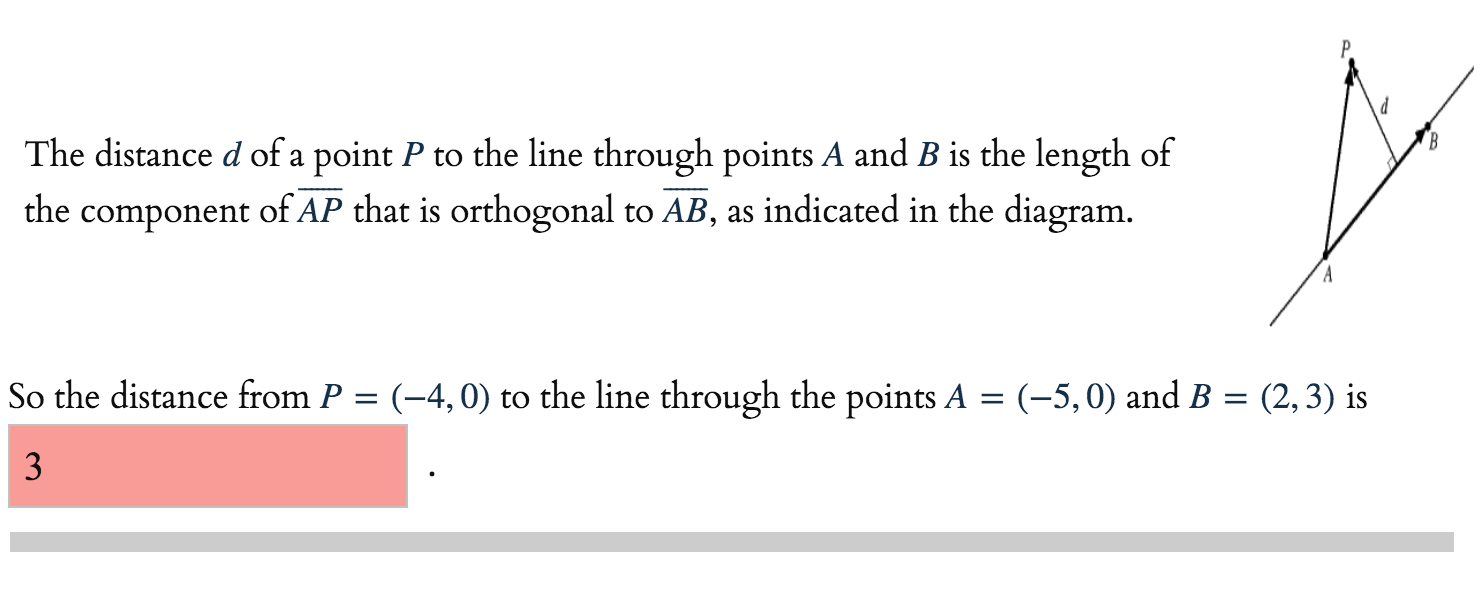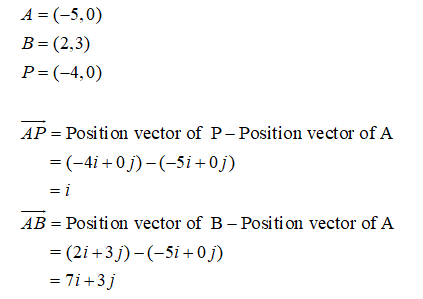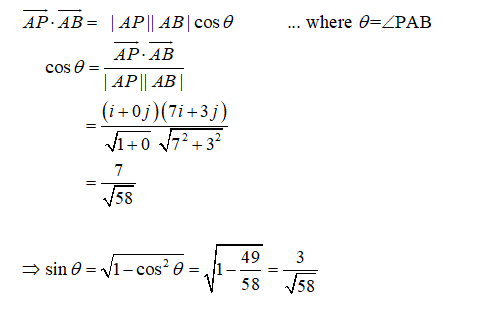# The distance d of a point P to the line through points A and B is the length ofthe component of AP that is orthogonal to AB, as indicated in the diagram.So the distance from P = (-4,0) to the line through the points A = (-5,0) and B = (2,3) is3

Question
1 viewshelp_outlineImage TranscriptioncloseThe distance d of a point P to the line through points A and B is the length of the component of AP that is orthogonal to AB, as indicated in the diagram. So the distance from P = (-4,0) to the line through the points A = (-5,0) and B = (2,3) is 3 fullscreen
check_circle

star
star
star
star
star
1 Rating
Step 1

The given points are,Step 2...

### Want to see the full answer?

See Solution

#### Want to see this answer and more?

Solutions are written by subject experts who are available 24/7. Questions are typically answered within 1 hour.*

See Solution
*Response times may vary by subject and question.
Tagged in

### Calculus